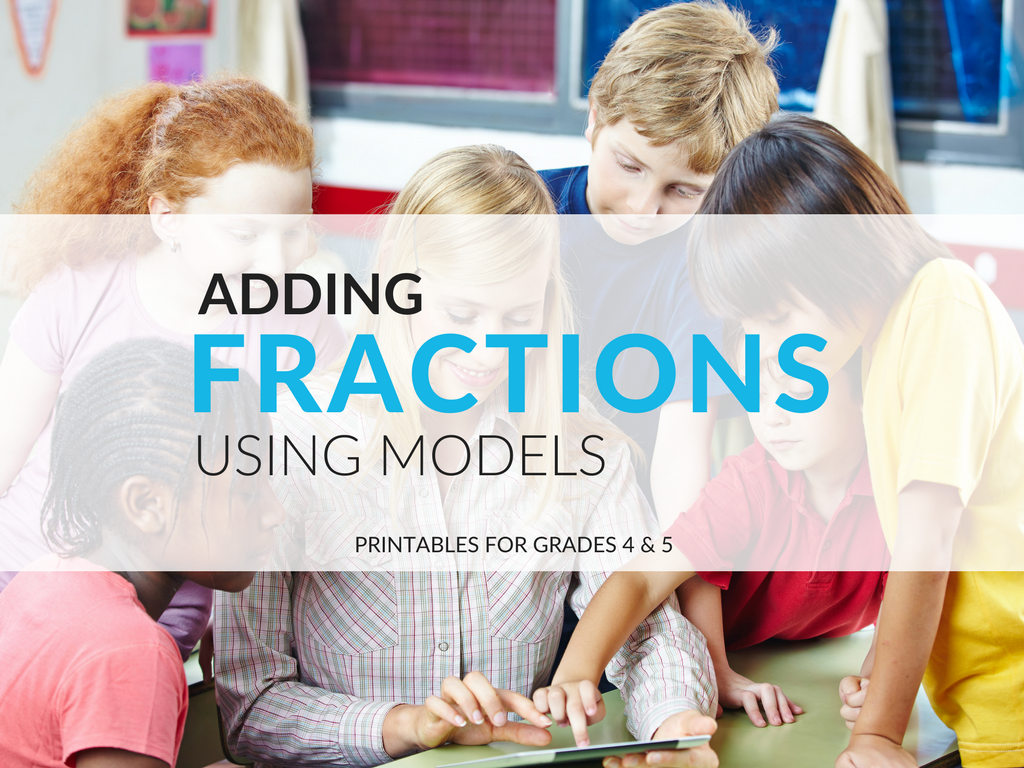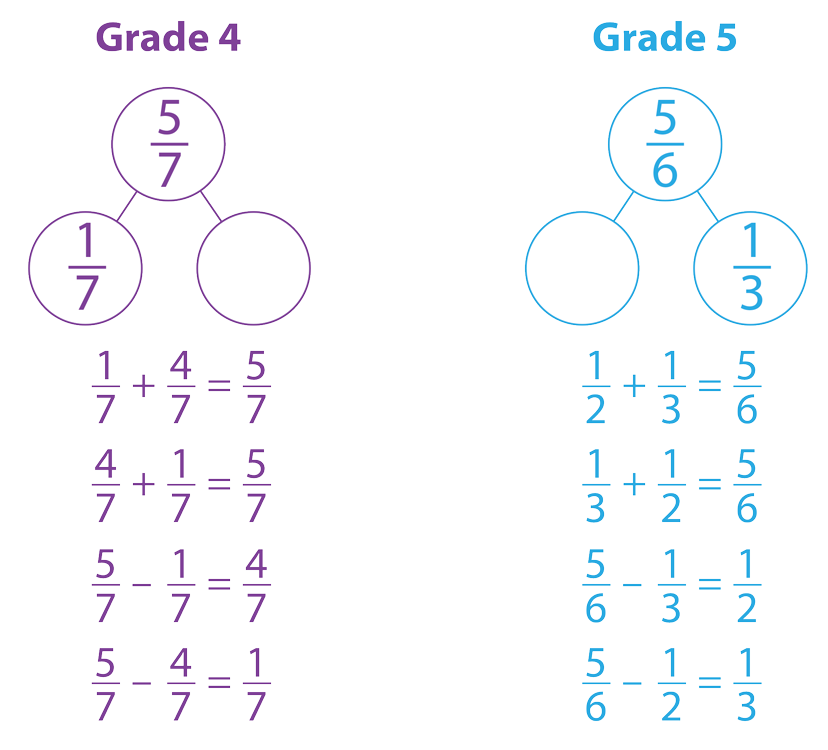Shop Now Login/Register View Quote View Cart
1.800.221.5175
Mathematics
Core Math
Full Access Progress in Mathematics Grades 1–6 View Details | Buy Now
Supplemental
Critical Thinking for Active Math Minds Grades 3–6 View Details | Buy Now
Vocabulary
Vocabulary Workshop, Tools for Comprehension Grades 1–5 View Details | Buy Now
Vocabulary Workshop, Tools for Comprehension Interactive Edition Grades 2–5 View Details | Buy Now
Vocabulary Workshop Achieve Interactive Edition Grades 6–12+ View Details | Buy Now
Vocabulary Workshop Enriched Edition Interactive Edition Grades 6–12+ View Details | Buy Now
Let's Target Vocabulary and Usage Grades 1–8 View Details | Buy Now
English Language Arts
Full Access Progress English Language Arts Grades 1–8 View Details | Buy Now
Grammar & Writing
Grammar Workshop, Tools for Writing Grades 3–5 View Details | Buy Now

A K–8 resource to support deep comprehension of math skills and concepts#### March 11, 2020 3-5-number-and-operations-fractions

This post and its related worksheets provide you with materials to help your students with visual representation of adding fractions using models, such as number lines and fraction strips.## Fraction Models Worksheets

Before we dive into the details of teaching students to add fractions using models, grab the two free worksheets I have available for download. For Progress in Mathematics users, these activities would align with Grade 4, Chapter 9 and Grade 5, Chapter 5. The adding fractions using models worksheets include an answer key!

## Adding Fractions On a Number Line

When I learned about adding fractions in elementary school, I remember that the instruction was more focused on numbers and rules, and less on using models to help me learn about the operations with fractions. But math teaching has changed a great deal since then, and it continues to evolve as we dig deeper into the Common Core State Standards for Mathematics.

Fractions can be pictorially represented in three ways:

1. With area models such as divided circles, squares, or rectangles

2. With array models using counters or dots arranged on a paper

3. With linear models, such as number strips or number lines

While the area and array models are used to develop the idea of equivalent fractions, number lines and fractions strips are used to help develop the idea of adding fractions. In Grade 3, students learn to create number lines marked with fractions. By Grades 4 and 5, the priority should be placed on teaching students to add fractions via models using number lines and fraction strips, rather than area or array models.

This use of number lines and measurement scales for adding fractions will be a key transferable skill for students. Students will extend their knowledge of addition to subtraction using number lines, and then to adding and subtracting rational numbers in Grade 7. In Grades 4 and 5, students will also construct line plots with a fractional measurement scale to collect data. Using two number lines placed at right angles, students learn about fraction multiplication using area models. The number lines at right angles are the basis of the coordinate system.

## Adding Fractions Using Number Bonds

One way I would extend the material in the downloadable fraction models worksheets is by having students show the relationships between the fractions using number bonds. Number bonds are another way to demonstrate adding fractions using models. In earlier grades, students used number bonds to show the relationship between whole numbers. Students should easily extend their knowledge of number bonds to the new domain of fractions. Once the number bond is formed, students should be able to write the four number sentences related to the bond. The table below shows an example from Grade 4 (same denominator) and Grade 5 (different denominators).Once they have learned to add fractions using number lines and number bonds, students address the measurement standards by solving word problems using these models. The word problems should include the topics of distance, time intervals, volumes of liquid, masses of objects, and money.

## In Summary

I hope these ideas about adding fractions with models have been helpful to you. If you need problems for students to solve using fraction bars, download the activity sheets, along with their answer keys.